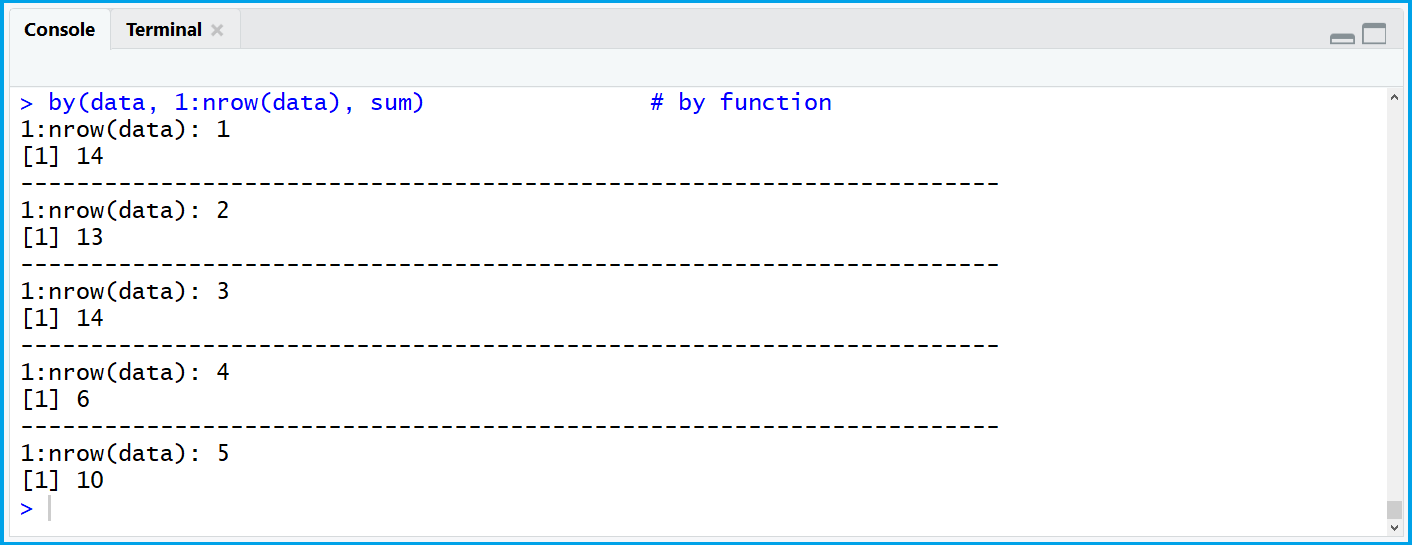# Use Function in Each Row of Data Frame in R (2 Examples)

In this article, I’ll show how to apply a function to each row of a data frame in the R programming language.

Let’s dive right into the examples:

## Constructing Example Data

First, we have to create some data that we can use in the examples later on. Consider the following data.frame:

```data <- data.frame(x1 = c(2, 6, 1, 2, 4),  # Create example data frame
x2 = c(7, 6, 5, 1, 2),
x3 = c(5, 1, 8, 3, 4))
data                                       # Inspect data in RStudio console
#  x1 x2 x3
#   2  7  5
#   6  6  1
#   1  5  8
#   2  1  3
#   4  2  4```

As you can see based on the RStudio console output, our data frame contains five rows and three numeric columns.

## Example 1: apply() Function

In Example 1, I’ll show you how to perform a function in all rows of a data frame based on the apply function. Let’s assume that our function, which we want to apply to each row, is the sum function.

Then, we can use the apply function as follows:

```apply(data, 1, sum)                        # apply function
# 14 13 14  6 10```

As you can see, the RStudio console returned the sum of each row – as we wanted.

## Example 2: by() Function

We can also use the by() function in order to perform a function within each row. We simply have to combine the by function with the nrow function:

`by(data, 1:nrow(data), sum)                # by function`Figure 1: Output of by() Function.

Figure 1 illustrates the RStudio console output of the by command. As you can see, the by function also returned the sum of each row, but this time in a readable format.

If you should prefer to use the apply function or the by function depends on your specific data situation.

## Video & Further Resources

Do you need more info on the content of this tutorial? Then you might have a look at the following video of my YouTube channel. In the video, I’m explaining the examples of this tutorial:

Please accept YouTube cookies to play this video. By accepting you will be accessing content from YouTube, a service provided by an external third party.If you accept this notice, your choice will be saved and the page will refresh.

Besides the video, you might read the other tutorials of www.statisticsglobe.com:

To summarize: In this article you learned how to repeat a function in each row without using a for-loop in the R programming language. Let me know in the comments, in case you have additional questions.

Subscribe to the Statistics Globe Newsletter